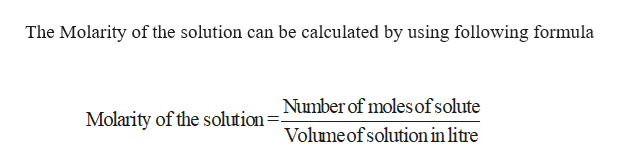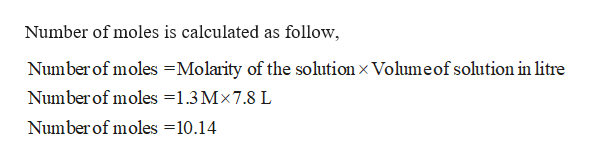# How many grams of glucose (C6H12O6) are present in 7.8 L of a 1.3 M glucose solution?

Question
41 views

How many grams of glucose (C6H12O6) are present in 7.8 L of a 1.3 M glucose solution?

check_circle

Step 1

Number of grams of glucose has to be calculated.

Given,

Volume = 7.8 L

Molarity = 1.3 M

Molar mass of glucose = 180.2 g/molhelp_outlineImage TranscriptioncloseThe Molarity of the solution can be calculated by using following formula Number of moles ofsolute Molarity of the solution Volumeof solution in litre fullscreen
Step 2

Number of moles of gluco...help_outlineImage TranscriptioncloseNumber of moles is calculated as follow Number of moles Molarity of the solution x Volume of solution in litre Number of moles =1.3 Mx7.8 L Number of moles 10.14 fullscreen

### Want to see the full answer?

See Solution

#### Want to see this answer and more?

Solutions are written by subject experts who are available 24/7. Questions are typically answered within 1 hour.*

See Solution
*Response times may vary by subject and question.
Tagged in

### General Chemistry